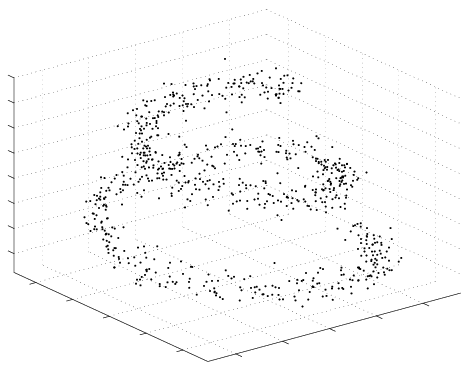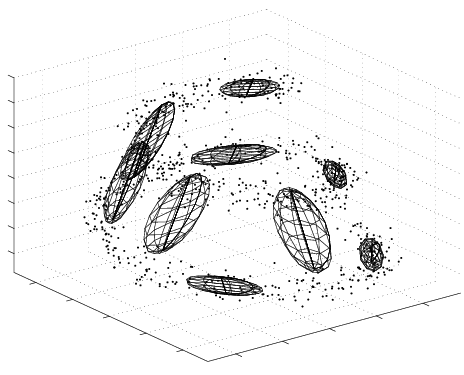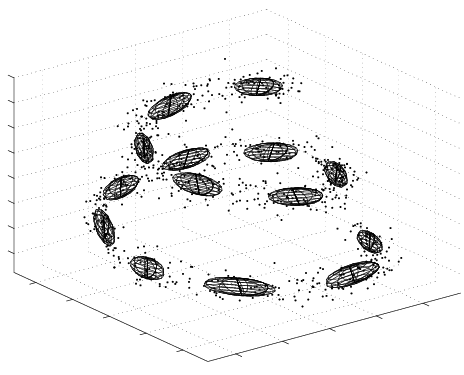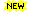I design machine learning algorithms that try to solve some of today's most challenging problems in computer science and statistics.

I adapt ideas from physics and the statistical sciences, and use them in algorithms that can be applied to areas such as: bioinformatics, artificial intelligence, pattern recognition, document information retrieval, and human-computer interaction.

Click on the following topics to see research descriptions and some papers:-

 Nonparametric Bayes - powerful nonparametric text/document modelling Variational Bayesian Methods - approximate Bayesian learning and inference Bioinformatics - microarray analysis using variational Bayes Embedded Hidden Markov Models - a novel tool for time series inference Probabilistic Sensor Fusion - combining modalities using Bayesian graphical models Collaborators - people I have worked with

### Variational Bayesian methods

Variational methods are a powerful tool from statistical physics that I have used to approximate Bayesian learning. Bayesian learning relies on a key quantity, the marginal likelihood, which results from averaging over the parameters of the model. In nearly every interesting scenario these averages are analytically intractable, and we are forced to work with approximations. Variational Bayesian methods offer a fast and efficient alternative to sampling techniques, and moreover offer an approximation in the form of a bound on the marginal likelihood.

My thesis presents a tutorial of variational methods, discusses and extends the theory behind the VB approximation, and applies it to several well-used algorithms in CS and statistics. These include models such as Mixtures of Factor Analysers, State-Space Models (Linear-Gaussian Dynamical Systems), and Hidden Markov Models.Example of a Variational Bayesian Mixture of Factor Analysers (VBMFA) discovering a non-linear 1-d manifold embedded in a 3-d space (the spiral). Iterations run from left (the data) to right (the final solution).

My work concentrates on a particular class of models called conjugate-exponential, which encompass the models described above, as well as a host of other models; I delve into these types of models and examine the variational Bayesian updates in detail. A number of key results are presented in the thesis. These include a general importance sampling methods for estimating the tightness of the VB lower bound. Also we show that the well-used Cheeseman-Stutz approximation to the marginal likelihood is not only itself a lower bound (similar to the VB lower bound), but also that there exists a straightforward construction which shows that the VB lower bound is universally tighter.

In the final chapter I show that VB outperforms standard model selection criteria, such as BIC and the Cheeseman-Stutz criterion, and comprehensively compare the VB performance to a gold-standard Annealed Importance Sampling (AIS) estimate. For a given accuracy we find that the VB algorithm needs less than 1% of the computational time that is required of the AIS sampling estimate.

•Beal, M.J.
Variational Algorithms for Approximate Bayesian Inference
PhD. Thesis, Gatsby Computational Neuroscience Unit, University College London, 2003.
•Beal, M.J., Ghahramani, Z.
The Variational Bayesian EM Algorithm for Incomplete Data: with Application to Scoring Graphical Model Structures
In Bayesian Statistics 7, Oxford University Press, 2003.
Preprint: [pdf] [ps.gz]. Conference proceedings preface [pdf] and contents [pdf]
• Ghahramani, Z. and Beal, M.J.
Propagation Algorithms for Variational Bayesian Learning
In Advances in Neural Information Processing Systems 13, eds. T.K. Leen, T. Dietterich, V. Tresp, MIT Press, 2001.
[pdf] [ps.gz] [poster] [software]
• Ghahramani, Z. and Beal, M.J.
Graphical Models and Variational Methods
Book chapter in Advanced Mean Field methods - Theory and Practice, eds. D. Saad and M. Opper, MIT Press. jacket
Part of this work is that discussed at NIPS*99 workshop of the same title as the book.
[pdf] [ps.gz]
• Ghahramani, Z. and Beal, M.J.
Variational Inference for Bayesian Mixtures of Factor Analysers
In Advances in Neural Information Processing Systems 12:449-455, eds. S. A. Solla, T.K. Leen, K. Müller, MIT Press, 2000.
[pdf] [ps.gz] [poster] [software]